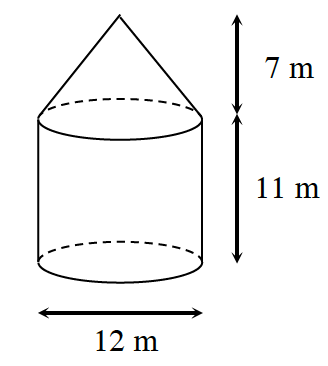### Home > CCG > Chapter 12 > Lesson 12.1.2 > Problem12-21

12-21.A silo (a structure designed to store grain) is designed as a cylinder with a cone on top, as shown in the diagram at right.

1. If a farmer wants to paint the silo, how much surface area must be painted?

Find the surface area of the cone, and the surface area of the cylinder.
Then find their sum.

The slant height of the cone $\approx9.22$ m
Lateral Area (cone) $=6\pi(9.22)\approx173.78$
Lateral Area (cylinder) $=12\pi(11)=132\pi\approx414.69$
$173.78+414.69=588.47m^2$

2. What is the volume of the silo? That is, how many cubic meters of grain can the silo hold?

Find the volume of the cone and the volume of the cylinder.
Then find their sum.